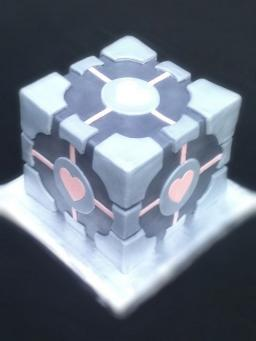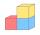# Wall diagonal

Calculate the length of wall diagonal of the cube whose surface is 384 cm square.

u =  11.3137 cm

### Step-by-step explanation:Did you find an error or inaccuracy? Feel free to write us. Thank you!Tips to related online calculators
Pythagorean theorem is the base for the right triangle calculator.

#### You need to know the following knowledge to solve this word math problem:

We encourage you to watch this tutorial video on this math problem:

## Related math problems and questions:

• Cube diagonalsThe cube has a wall area of 81 cm square. Calculate the length of its edge, wall, and body diagonal.
• Cube wallThe perimeter of one cube wall is 120 meters. Calculate the surface area and the body diagonal of this cube.
• Cube volumeThe cube has a surface of 384 cm2. Calculate its volume.
• Cube wallCalculate the cube's diagonal if you know that one wall's surface is equal to 36 centimeters square. Please also calculate its volume.
• CalculateCalculate the length of the wall diagonal of the cube with an edge 5 cm long.
• Surface and volume - cubeFind the surface and volume of a cube whose wall diagonal is 5 cm long.
• Cube diagonalsDetermine the volume and surface area of the cube if you know the length of the body diagonal u = 216 cm.
• Body diagonal - cubeCalculate the surface and cube volume with body diagonal 15 cm long.
• Cube and sphereCube with the surface area 150 cm2 is described sphere. What is sphere surface?
• Cube wallsFind the volume and surface area of the cube if the area of one wall is 40cm2.
• The cubeThe cube has a surface area of 216 dm2. Calculate: a) the content of one wall, b) edge length, c) cube volume.
• Regular square prismThe volume of a regular square prism is 192 cm3. The size of its base edge and the body height is 1: 3. Calculate the surface of the prism.
• Square diagonalCalculate the length of the square diagonal if the perimeter is 172 cm.
• A squareA square with a length of diagonals 12cm give: a) Calculate the area of a square b) rhombus with the same area as the square, has one diagonal with a length of 16 cm. Calculate the length of the other diagonal.Calculate the volume and surface area of a regular quadrangular prism 35 cm high and the base diagonal of 22 cm.Calculate the surface of the cube ABCDA'B'C'D' if the area of rectangle ACC'A' = 344 mm2.The surface of the first cube wall is 64 m2. The second cube area is 40% of the surface of the first cube. Determine the length of the edge of the second cube (x).